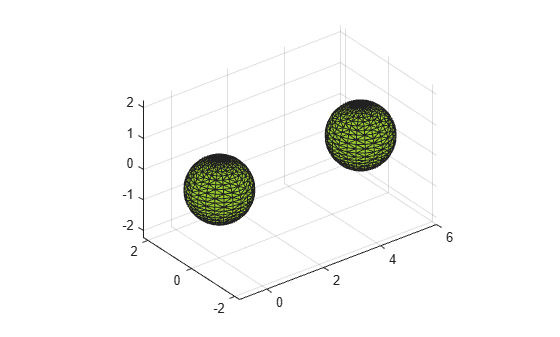# plot

Plot alpha shape

## Syntax

``plot(shp)``
``plot(shp,Name,Value)``
``h = plot(___)``

## Description

example

````plot(shp)` plots alpha shape `shp` in a figure window.```

example

````plot(shp,Name,Value)` uses additional options specified by one or more `Name,Value` pair arguments. For a complete list of allowed `Name,Value` pairs, see Patch Properties.```
````h = plot(___)` returns a handle to a `Patch` object using any of the previous syntaxes.```

## Examples

collapse all

Create a set of 3-D points.

```[x1, y1, z1] = sphere(24); x1 = x1(:); y1 = y1(:); z1 = z1(:); x2 = x1+5; P = [x1 y1 z1; x2 y1 z1]; P = unique(P,'rows');```

Create and plot an alpha shape using an alpha radius of 1.5.

```shp = alphaShape(P,1.5); plot(shp)```Plot the alpha shape with a specified color and transparency factor.

`plot(shp,'FaceColor','red','FaceAlpha',0.1)`## Input Arguments

collapse all

Alpha shape, specified as an `alphaShape` object. For more information, see `alphaShape`.

Example: `shp = alphaShape(x,y)` creates a 2-D `alphaShape` object from the `(x,y)` point coordinates.

## Output Arguments

collapse all

Handle to `Patch` object, returned as a handle. For more information, see Introduction to Patch Objects.

## Version History

Introduced in R2014b